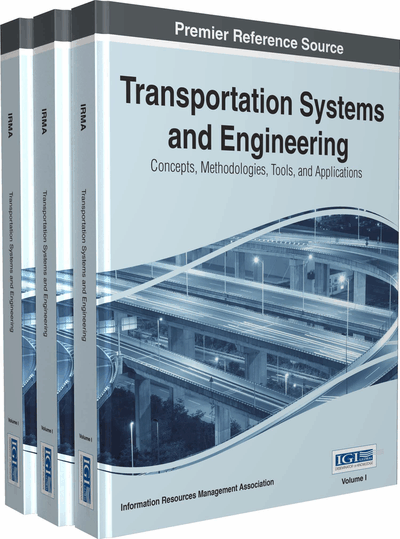# GeneticTKM: A Hybrid Clustering Method Based on Genetic Algorithm, Tabu Search and K-Means

Masoud Yaghini (Iran University of Science and Technology, Iran) and Nasim Gereilinia (Iran University of Science and Technology, Iran)
DOI: 10.4018/978-1-4666-8473-7.ch033
Available
\$37.50
No Current Special Offers

## Abstract

The clustering problem under the criterion of minimum sum square of errors is a non-convex and non-linear problem, which possesses many locally optimal values, resulting that its solution often being stuck at locally optimal solution. In this paper, a hybrid genetic, tabu search and k-means algorithm, called GeneticTKM, is proposed for the clustering problem. A new mutation operator is presented based on tabu search algorithm for the proposed hybrid genetic method. The key idea of the new operator is to produce tabu space for escaping from trap of local optimal and finding better solution. The results of the proposed algorithm are compared with other clustering algorithms such as genetic algorithm; tabu search and particle swarm optimization by implementing them and using standard and simulated data sets. The authors also compare the results of the proposed algorithm with other researchers' results in clustering the standard data sets. The results show that the proposed algorithm can be considered as an effective and efficient algorithm to find better solution for the clustering problem.
Chapter Preview
Top

## 1. Introduction

The clustering is the process of grouping a set of objects into classes (Han & Kamber, 2006). The objects in the same cluster are similar to one another and are dissimilar to the objects in other clusters. k-means is one of the popular clustering methods. This algorithm divides a set of n objects in to k clusters so that the resulting intracluster similarity is high but the intercluster similarity is low. The k-means algorithm proceeds as follows. First, it randomly selects k of the objects as cluster centers. For each of the remaining objects, an object is assigned to the cluster to which it is the most similar, based on the distance between the object and the cluster center. It then computes the new cluster centers as mean value of the objects in each cluster. The above process continues until the criterion function converges. Usually, the square-error criterion is used. It is calculated as follows:

(1) where F is the sum of the square error for all objects in the data set; p is the point in space representing a given object; and mi is the mean of cluster Ci. In other words, the distances between objects and their cluster centers are squared and then summed (Han & Kamber, 2006). The major drawback of k-means algorithm is that the clustering quality is sensitive to the initial election of cluster centers and may converge to the local optimal (Abdel-Kader, 2010).

In recent years, the various metaheuristic algorithms such as genetic algorithm and tabu search are used to avoid getting trapped in local optimal solutions in solving the clustering problem. In domain of genetic algorithm, a genetically based algorithm, which is composed of split and merge algorithm proposed in Garai and Chaunhuri (2004). Laszlo and Mukherjee (2006) presented a genetic algorithm to select a good set of centers for k-means to produce near optimal partitions. Their approach uses hyper-quad trees. The main drawback of this algorithm is that it is only suitable for low-dimensional data, so Laszlo and Mukherjee (2007) presented another method that exploits region-based crossover, but unlike the hyper-quad tree approach, it scales well to higher-dimensional data sets. Hong & Kwong (2008) proposed a novel clustering algorithm that combines the steady-state genetic algorithm and the ensemble learning method. Chang et al. (2009) suggested a Genetic Algorithm with Gene Rearrangement (GAGR). In their algorithm, in order to reduce the degeneracy, a gene rearrangement of the chromosome has been used. Degeneracy occurs when different chromosomes showing the same cluster result. Moreover, a new path-based crossover operator has been presented. This operator builds a path from one chromosome to another chromosome.

## Complete Chapter List

Search this Book:
Reset